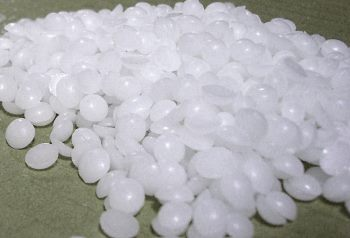calculator.org

# property>molar heat capacity

## What is Molar Heat Capacity?The Molar Heat Capacity is just like the specific heat capacity in Chemistry, except its units differ. A specific heat capacity is the amount of energy necessary to increase the temperature of a kilogram of that substance by one kelvin. Molar heat capacity is the amount of energy necessary to raise one mole of substance by one kelvin degree. One mole of a substance is exactly 6.02x1023 atoms or molecules of that substance. If we know how many kilograms we have of a substance, and we also know the atomic weight of that substance, we can find out how many moles we have of the substance. We can therefore convert the specific heat capacity of a kilogram of a substance to the molar heat capacity in the following way:

Cn = Cp*mmole

where Cn is the molar heat capacity of the substance, Cp is the specific heat, and mmole is the mass per mole of the substance, which is essentially the atomic weight (measured in g/mol). The SI units for molar heat capacity are joules/(mole.kelvin).

The equation relating the heat energy Q to molar heat and a temperature change work in a slightly different way than those for specific heat capacity. This equation is written as:

Q = n.c.ΔT

where n is the number of moles of the substance, c is the molar heat capacity, and ΔT is the change in temperature. One can also derive the molar heat capacity by rearranging the same equation such that:

c = Q/(n.ΔT)

Almost all values of molar heat will stay constant over a wide temperature range, except for very very low temperatures. This is where the laws of quantum mechanics begin to take precedence over what would ordinarily be classical behaviour. The Einstein-Debye model is able to predict the temperature dependence of the molar heat capacity for these very low temperatures. It assumes that heat is made up of vibrations in an atomic lattice and then treats it as if it is also phonons in a box.

Certain sorts of compounds are more likely than others to have a high molar heat capacity due to varying degrees of freedom of motion present in these molecules. A more complex molecule composed of many bonds will have more degrees of freedom than a simpler version with less bonds. Within these additional degrees of freedom more heat energy in the form of vibrations can be stored. Interestingly, there are cases in which the addition of heat to a complex molecule will actually assist in the opening up of more degrees of freedom, thus increasing its molar heat capacity. This is known to occur with nitrogen which has five degrees of freedom at room temperature but gains two more internal degrees of freedom at higher temperatures. This, in turn, causes its molar heat capacity to increase.

Paraffin wax has a good specific heat capacity for use as a heat storage medium (an example application being heated hair curlers) and also has a very high molar heat capacity by virtue of it's high molecular weight.

Bookmark this page in your browser using Ctrl and d or using one of these services: (opens in new window)